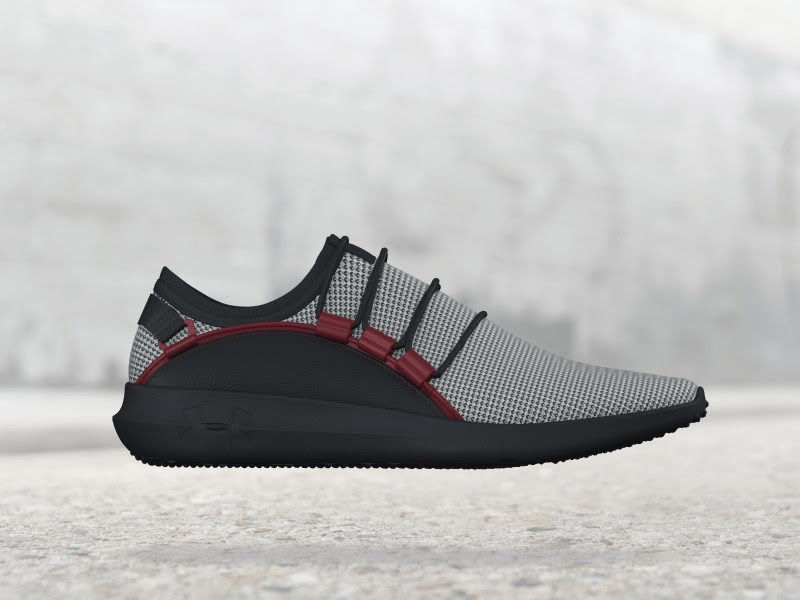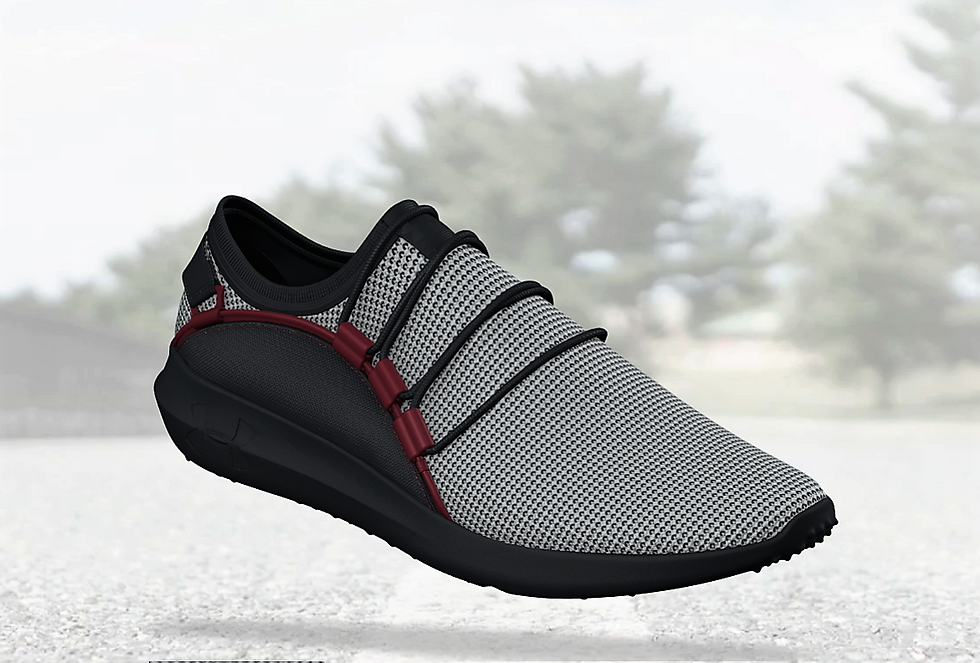top of page
Search

# The Eye-Slider Shoehis is the way. This is the way. This is the way. This is the way. his is the way. This is the way. This is the way. This is the way. his is the way. This is the way. This is the way. This is the way. his is the way. This is the way. This is the way. This is the way. his is the way. This is the way. This is the way. This is the way.his is the way. This is the way. This is the way. This is the way. his is the way. This is the way. This is the way. This is the way. his is the way. This is the way. This is the way. This is the way. his is the way. This is the way. This is the way. This is the way. his is the way. This is the way. This is the way. This is the way.